# Image Pyramids¶

Faisal Qureshi
Professor
Faculty of Science
Ontario Tech University
http://vclab.science.ontariotechu.ca

## Lesson Plan¶

• Gaussian image pyramids
• Laplacian image pyramids
• Laplacian blending

## Uses¶

Often used to achieve scale-invariant processing in the following contexts:

• template matching;
• image registration;
• image enhancement;
• interest point detection; and
• object detection.

## Gaussian Image Pyramid¶

The basic idea for constructing Gaussian image pyramid is as follows:

1. Gaussian blur the image
2. Reduce image dimensions by half by discarding every other row and and every other column
3. Repeat this process until desired numbers levels are achieved or the image is reduced to size $1 \times 1$.
In :
import numpy as np
import cv2 as cv
import matplotlib.pyplot as plt


## Exercise 04-01¶

• Blur each channel with a 5-by-5 Gaussian kernel
• Construct the next level of Gaussian pyramid by discarding every other row or column
In :
# %load solutions/image-pyramids/solution_01.py
I = cv.cvtColor(I, cv.COLOR_BGR2RGB)
I = cv.resize(I, (512, 512))

print('Shape of I = {}'.format(I.shape))

I[:,:,0] = cv.GaussianBlur(I[:,:,0], (5,5), 2, 2)
I[:,:,1] = cv.GaussianBlur(I[:,:,1], (5,5), 2, 2)
I[:,:,2] = cv.GaussianBlur(I[:,:,2], (5,5), 2, 2)

I2 = I[::2,::2,:]
print('Shape of I2 = {}'.format(I2.shape))

plt.imshow(I2)

Shape of I = (512, 512, 3)
Shape of I2 = (256, 256, 3)

Out:
<matplotlib.image.AxesImage at 0x12e4cdcd0>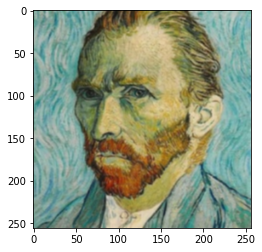## Implementation (Gaussian Image Pyramid)¶

In :
# %load solutions/image-pyramids/gen_gaussian_pyramid.py
def gen_gaussian_pyramid(I, levels=6):
G = I.copy()
gpI = [G]
for i in range(levels):
G = cv.pyrDown(G)
gpI.append(G)
return gpI

In :
# %load solutions/image-pyramids/gen_gaussian_pyramid.py
def gen_pyramid(I, levels=6):
G = I.copy()
pI = [G]
for i in range(levels):
G = G[::2,::2,:]
pI.append(G)
return pI

In :
foo = gen_gaussian_pyramid(I, levels=9)
boo = gen_pyramid(I, levels=9)

for i in foo:
print(i.shape)

for i in boo:
print(i.shape)

In :
plt.figure(figsize=(10,5))
plt.subplot(121)
plt.imshow(boo)
plt.subplot(122)
plt.imshow(foo)

Out:
<matplotlib.image.AxesImage at 0x12f143c50>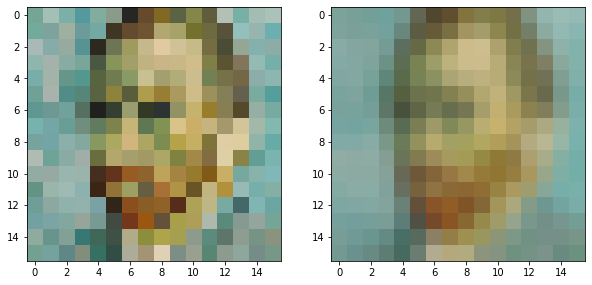In :
plt.figure(figsize=(2,1))
plt.subplot(121)
plt.imshow(cv.resize(boo, (512,512)))
plt.subplot(122)
plt.imshow(cv.resize(foo, (512,512)))

Out:
<matplotlib.image.AxesImage at 0x130470790>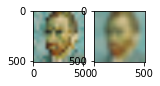OpenCV pyrDown method performs steps 1 and 2 above. It uses the following kernel to blur the image (in step 1).

$$\frac{1}{256} \left[ \begin{array}{ccccc} 1 & 4 & 6 & 4 & 1 \\ 4 & 16 & 24 & 16 & 4 \\ 6 & 24 & 36 & 24 & 6 \\ 4 & 16 & 24 & 16 & 4 \\ 1 & 4 & 6 & 4 & 1 \end{array} \right]$$
In :
A = cv.imread('data/apple.jpg')
A = cv.cvtColor(A, cv.COLOR_BGR2RGB)
B = cv.cvtColor(B, cv.COLOR_BGR2RGB)

In :
gpA = gen_gaussian_pyramid(A)
gpB = gen_gaussian_pyramid(B)

In :
gp = gpB
num_levels = len(gp)
for i in range(num_levels):
rows = gp[i].shape
cols = gp[i].shape
print('level={}: size={}x{}'.format(i, rows, cols))

level=0: size=512x512
level=1: size=256x256
level=2: size=128x128
level=3: size=64x64
level=4: size=32x32
level=5: size=16x16
level=6: size=8x8

In :
gp = gpA
level = 3
plt.figure(figsize=(10,10))
plt.title('Level {}'.format(level))
plt.imshow(gp[level])

Out:
<matplotlib.image.AxesImage at 0x1305cfc50>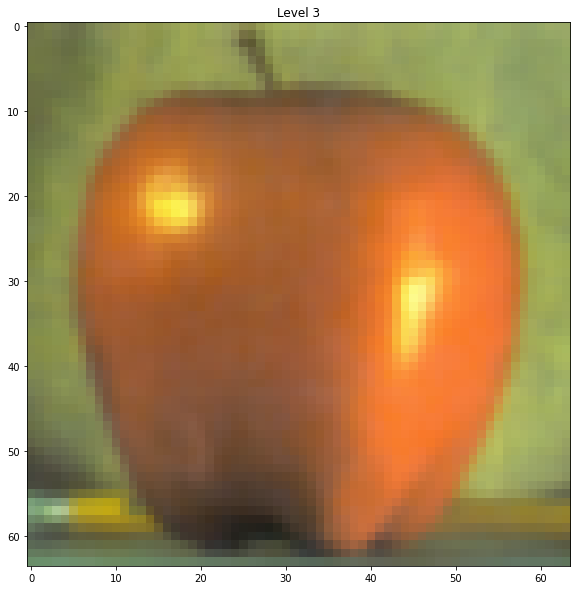## Laplacian Image Pyramid¶

Proposed by Burt and Adelson is a bandpass image decomposition derived from the Gaussian pyramid. Each level encodes information at a particular spatial frequency. The basic steps for constructing Laplacian pyramids are:

1. Convolve the original image $g_0$ with a lowpass filter $w$ (e.g., the Gaussian filter) and subsample it by two to create a reduced lowpass version of the image $g_1$. Recall that this is what function pyrDown does.

2. Upsample this image (i.e., $g_1$) by inserting zeros in between each row and column and interpolating the missing values by convolving it with the same filter $w$ to create the expanded lowpass image $g'_1$ which is subtracted pixel by pixel from the original to give the detail image $L_0$. Specifically $L_0 = g_0 - g'_1$.

3. Repeat steps 1 and 2.

We define Laplacian operator as follows:

$$\nabla^2 f = \frac{\partial^2 f}{\partial x^2} + \frac{\partial^2 f}{\partial y^2}$$

In addition we can approximate the Laplacian of a Gaussian as follows:

We use this property when constructing Laplacian image pyramids above.

### Reconstructing the original image¶

It is possible to reconstruct the original image $g_0$ from its Laplacian image pyramd consisting of $N+1$ detail images $L_i$, where $i \in [0,N]$ and the low pass image $g_N$.

1. $g_N$ is upsampled by inserting zeros between the sample values and interpolating the missing values by convolving it with the filter $w$ to obtain the image $g'_N$.
2. The image $g'_N$ is added to the lowest level detail image $L_N$ to obtain the approximation image at the next upper level.
3. Steps 1 and 2 are repeated on the detail images $L_i$ to obtain the original image.

### Uses¶

Laplacian image pyramids are often used for compression. Instead of encoding $g_0$, we encode $L_i$, which are decorrelated and can be represented using far fewer bits.

## Implementation (Laplacian Image Pyramid)¶

In :
# %load solutions/image-pyramids/gen_laplacian_pyramid.py
def gen_laplacian_pyramid(gpI):
"""gpI is a Gaussian pyramid generated using gen_gaussian_pyramid method found in py file of the same name."""
num_levels = len(gpI)-1
lpI = [gpI[num_levels]]
for i in range(num_levels,0,-1):
GE = cv.pyrUp(gpA[i])
L = cv.subtract(gpA[i-1],GE)
lpI.append(L)
return lpI

In :
lpA = gen_laplacian_pyramid(gpA)
lpB = gen_laplacian_pyramid(gpB)

In :
lp = lpA
num_levels = len(lp)
for i in range(num_levels):
rows = lp[i].shape
cols = lp[i].shape
print('level={}: size={}x{}'.format(i, cols, rows))

level=0: size=8x8
level=1: size=16x16
level=2: size=32x32
level=3: size=64x64
level=4: size=128x128
level=5: size=256x256
level=6: size=512x512

In :
plt.imshow(lp)

Out:
<matplotlib.image.AxesImage at 0x13142a9d0>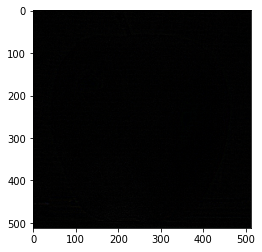## Laplacian Blending Example¶

In :
lp = lpA
level = 3
plt.figure(figsize=(10,10))
plt.title('Level {}'.format(level))
plt.imshow(lp[level])

Out:
<matplotlib.image.AxesImage at 0x131491c50>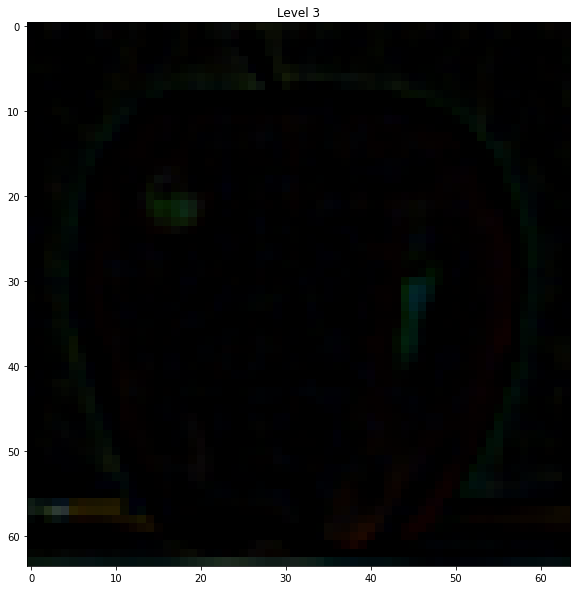In :
# %load solutions/image-pyramids/laplacian_blending.py
LS = []
for la, lb in zip(lpA, lpB):
rows, cols, dpt = la.shape
ls = np.hstack((la[:,0:cols//2,:], lb[:,cols//2:,:]))
LS.append(ls)

In :
# %load solutions/image-pyramids/reconstruct_from_laplacian.py
ls_ = LS
for i in range(1,6):
ls_ = cv.pyrUp(ls_)

In :
real = np.hstack((A[:,:cols//2],B[:,cols//2:]))

In :
plt.figure(figsize=(10,10))
plt.imshow(ls_)

Out:
<matplotlib.image.AxesImage at 0x13166b2d0>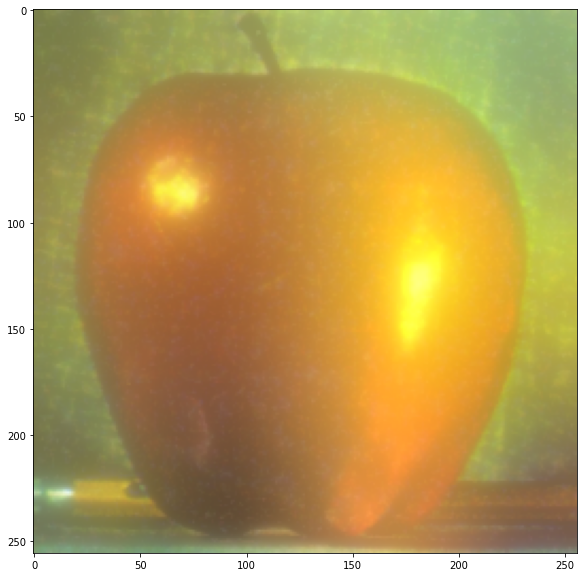In :
plt.figure(figsize=(10,10))
plt.imshow(real)

Out:
<matplotlib.image.AxesImage at 0x12f730490>